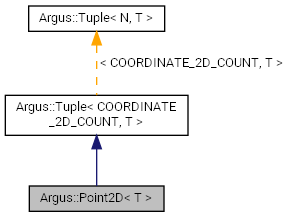## L4T Multimedia API Reference

#### 32.2 Release

Argus::Point2D< T > Class Template Reference

## Detailed Description

### template<typename T> class Argus::Point2D< T >

Point2D template class.

This is a Tuple specialization containing 2 elements corresponding to the x and y coordinates a 2D point. Values can be accessed using the named methods or subscript indexing using the Argus::Coordinate enum.

Definition at line 607 of file Types.h.

Inheritance diagram for Argus::Point2D< T >:[legend]
Collaboration diagram for Argus::Point2D< T >:[legend]

## Public Member Functions

Point2D ()

Point2D (const Tuple< COORDINATE_2D_COUNT, T > &other)

Point2D (T init)

Point2D (T _x, T _y)

T & x ()

const T & x () const

T & y ()

const T & y () const

bool operator== (const Tuple< N, T > &rhs) const
Returns true when every element in the two tuples are identical. More...

bool operator!= (const Tuple< N, T > &rhs) const
Returns true if there are any differences between the two tuples. More...

Tuple< N, T > & operator+= (const Tuple< N, T > &rhs)
Adds every element of another tuple to the elements of this tuple. More...

Tuple< N, T > & operator-= (const Tuple< N, T > &rhs)
Subtracts every element of another tuple from the elements of this tuple. More...

Tuple< N, T > & operator*= (const T &rhs)
Multiplies every element in the tuple by a single value. More...

Tuple< N, T > & operator/= (const T &rhs)
Divides every element in the tuple by a single value. More...

const Tuple< N, T > operator+ (const Tuple< N, T > &rhs) const
Returns the result of adding another tuple to this tuple. More...

const Tuple< N, T > operator- (const Tuple< N, T > &rhs) const
Returns the result of subtracting another tuple from this tuple. More...

const Tuple< N, T > operator* (const T &rhs) const
Returns the result of multiplying this tuple by a single value. More...

const Tuple< N, T > operator/ (const T &rhs) const
Returns the result of dividing this tuple by a single value. More...

T & operator[] (unsigned int i)

const T & operator[] (unsigned int i) const

## Static Public Member Functions

static unsigned int tupleSize ()
Returns the number of elements in the tuple. More...

m_data [N]

## Constructor & Destructor Documentation

template<typename T>
 Argus::Point2D< T >::Point2D ( )
inline

Definition at line 610 of file Types.h.

template<typename T>
 Argus::Point2D< T >::Point2D ( const Tuple< COORDINATE_2D_COUNT, T > & other )
inline

Definition at line 611 of file Types.h.

template<typename T>
 Argus::Point2D< T >::Point2D ( T init )
inline

Definition at line 613 of file Types.h.

References Argus::Point2D< T >::x(), and Argus::Point2D< T >::y().

template<typename T>
 Argus::Point2D< T >::Point2D ( T _x, T _y )
inline

Definition at line 618 of file Types.h.

References Argus::Point2D< T >::x(), and Argus::Point2D< T >::y().

## Member Function Documentation

 bool Argus::Tuple< N, T >::operator!= ( const Tuple< N, T > & rhs ) const
inlineinherited

Returns true if there are any differences between the two tuples.

Definition at line 463 of file Types.h.

 const Tuple Argus::Tuple< N, T >::operator* ( const T & rhs ) const
inlineinherited

Returns the result of multiplying this tuple by a single value.

Definition at line 513 of file Types.h.

 Tuple& Argus::Tuple< N, T >::operator*= ( const T & rhs )
inlineinherited

Multiplies every element in the tuple by a single value.

Definition at line 485 of file Types.h.

 const Tuple Argus::Tuple< N, T >::operator+ ( const Tuple< N, T > & rhs ) const
inlineinherited

Returns the result of adding another tuple to this tuple.

Definition at line 501 of file Types.h.

 Tuple& Argus::Tuple< N, T >::operator+= ( const Tuple< N, T > & rhs )
inlineinherited

Adds every element of another tuple to the elements of this tuple.

Definition at line 469 of file Types.h.

 const Tuple Argus::Tuple< N, T >::operator- ( const Tuple< N, T > & rhs ) const
inlineinherited

Returns the result of subtracting another tuple from this tuple.

Definition at line 507 of file Types.h.

 Tuple& Argus::Tuple< N, T >::operator-= ( const Tuple< N, T > & rhs )
inlineinherited

Subtracts every element of another tuple from the elements of this tuple.

Definition at line 477 of file Types.h.

 const Tuple Argus::Tuple< N, T >::operator/ ( const T & rhs ) const
inlineinherited

Returns the result of dividing this tuple by a single value.

Definition at line 519 of file Types.h.

 Tuple& Argus::Tuple< N, T >::operator/= ( const T & rhs )
inlineinherited

Divides every element in the tuple by a single value.

Definition at line 493 of file Types.h.

 bool Argus::Tuple< N, T >::operator== ( const Tuple< N, T > & rhs ) const
inlineinherited

Returns true when every element in the two tuples are identical.

Definition at line 457 of file Types.h.

 T& Argus::Tuple< N, T >::operator[] ( unsigned int i )
inlineinherited

Definition at line 524 of file Types.h.

 const T& Argus::Tuple< N, T >::operator[] ( unsigned int i ) const
inlineinherited

Definition at line 525 of file Types.h.

 static unsigned int Argus::Tuple< N, T >::tupleSize ( )
inlinestaticinherited

Returns the number of elements in the tuple.

Definition at line 528 of file Types.h.

template<typename T>
 T& Argus::Point2D< T >::x ( )
inline

Definition at line 624 of file Types.h.

References Argus::COORDINATE_X.

Referenced by Argus::Array2D< T >::operator()(), and Argus::Point2D< T >::Point2D().

template<typename T>
 const T& Argus::Point2D< T >::x ( ) const
inline

Definition at line 625 of file Types.h.

References Argus::COORDINATE_X.

template<typename T>
 T& Argus::Point2D< T >::y ( )
inline

Definition at line 626 of file Types.h.

References Argus::COORDINATE_Y.

Referenced by Argus::Array2D< T >::operator()(), and Argus::Point2D< T >::Point2D().

template<typename T>
 const T& Argus::Point2D< T >::y ( ) const
inline

Definition at line 627 of file Types.h.

References Argus::COORDINATE_Y.

## Field Documentation

 T Argus::Tuple< N, T >::m_data[N]
protectedinherited

Definition at line 531 of file Types.h.

The documentation for this class was generated from the following file: